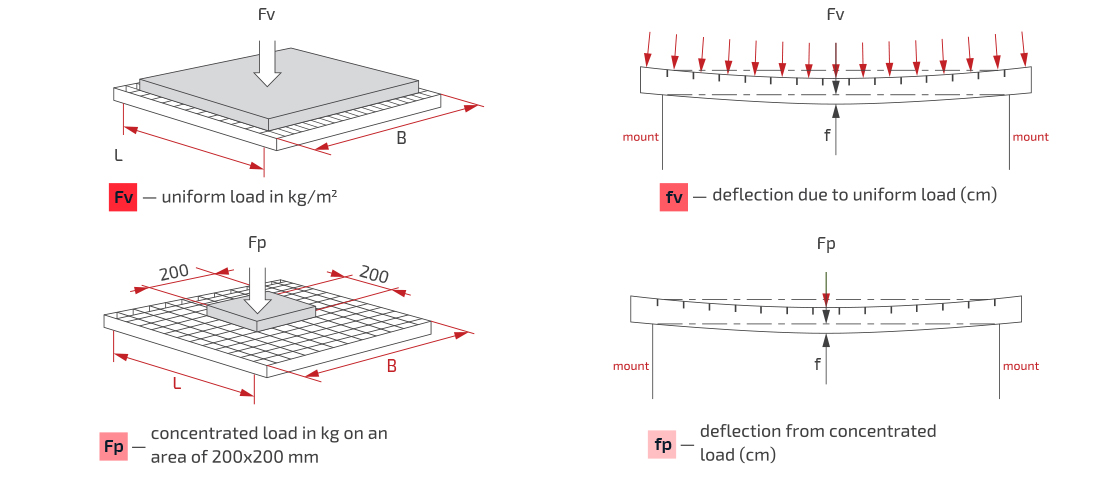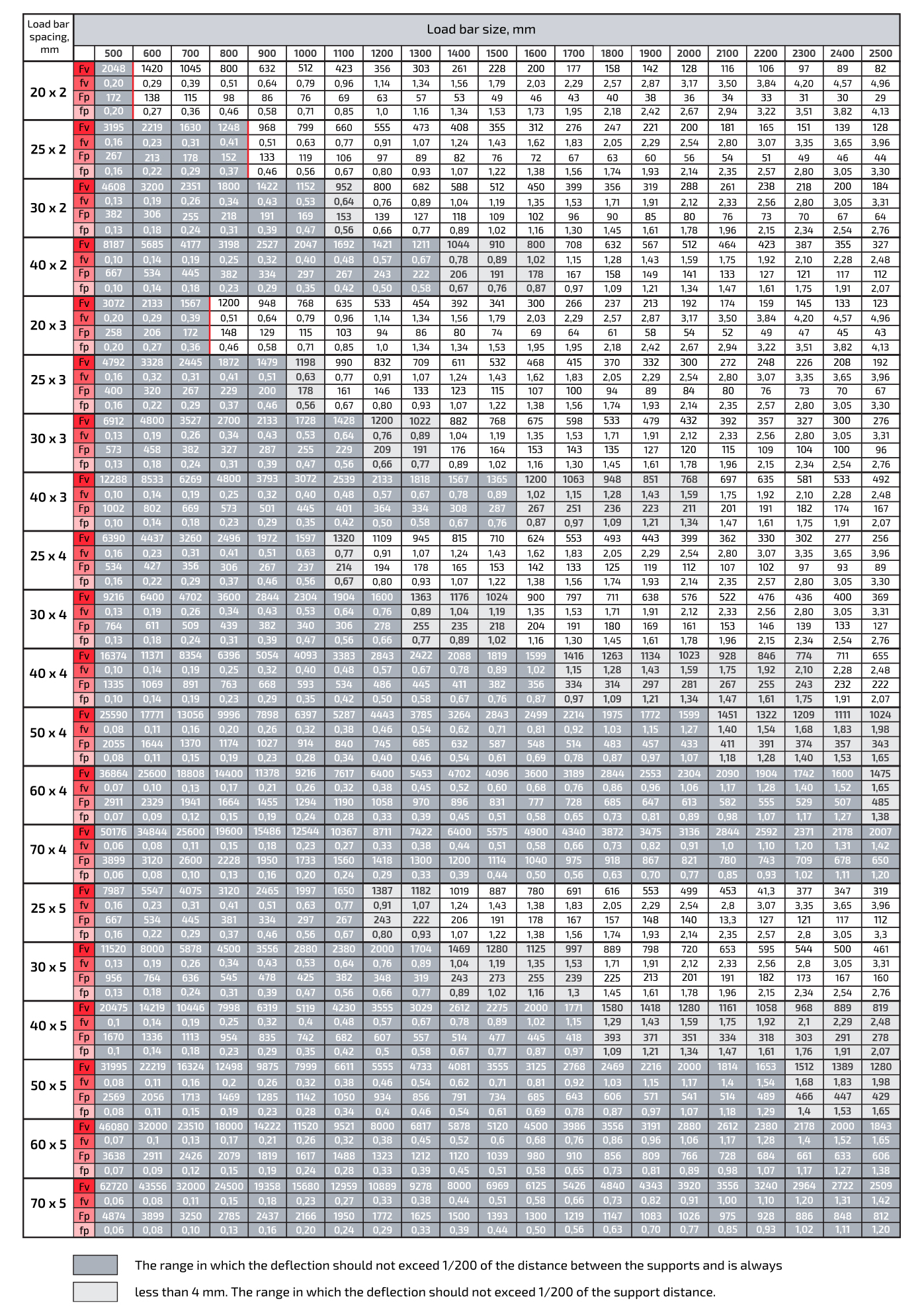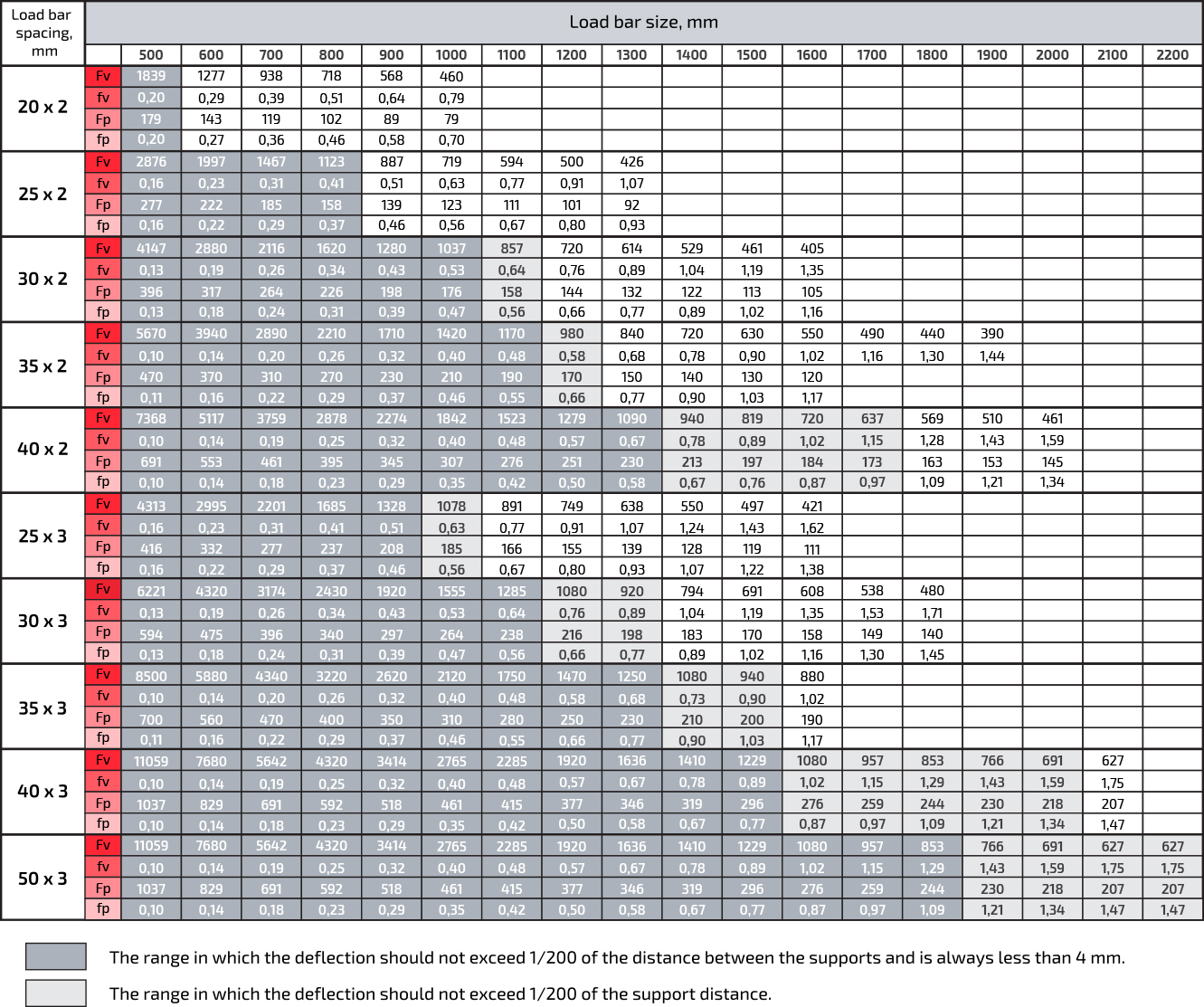en
Call back

# How to calculate the load on the grating

The gratings have an excellent load-bearing capacity and low own weight. This makes it unique and optimal solution for the construction of metal structures for high loads. We tried to show as clearly as possible what kind of load welded and pressed gratings can bear.

• Distributed load Fv — the maximum weight that is applied simultaneously over the entire plane of the grating.
• Concentrated load Fp — weight, applied on a plane of 200x200 mm in any place of the grating.
• Deflection from concentrated load — how much the grating will bend when exposed to load.### Welded grating – load table#### How to calculate the load on welded gratings of other sizes

The data from the table are given for gratings with a standard size of cell 34x38 mm. Cells are also available in sizes 34x50, 34x76, 34x101 mm. To calculate the load for such gratings, it is enough to multiply the Fv value from the table by a factor of 0.95.

##### An example of calculating the permissible load

We need to find the maximum permissible uniform load on a cell with a bearing strip of 40x3 mm, a pitch of 1000 mm and a cell size of 34.3x76.2 mm.

1. We take the Fv value from the table – 3072 kg/m².
2. Coefficient for cell 34.3x76.2 mm – 0.95.
3. Multiply two numbers 3072 * 0.95 = 2918 kg/m².

### Press grating – load table##### How to calculate the load on the pressed gratings of other sizes

The table shows the loads for lattices with a distance between the bearing strips of 33.3 mm. To calculate the permissible loads for grilles with other parameters, use the table of raising and lowering factors, which is presented below↓.

 Load bar spacing, mm The number of bearing bars 1 m width Coefficient, w 11,11 91 2,93 22,22 11,11 1,48 33,33 31 1 44,44 23 0,74 55,55 19 0,61 66,66 6 0,52
###### An example of calculating the load on a non-standard grating

Calculate the maximum uniform load Fv for a grid with a bearing strip spacing of 44 mm and a support spacing of 800 mm.

1. According to the load table, the Fv value for such a deck is 2430 kg/m².
2. Coefficient for 44 mm cell – 0.74.
3. We multiply two numbers, and we get: 2430 x 0.74 = 1798 kg/m².

If you need a grating of non-standard dimensions, then the permissible loads for it are calculated individually. To do this, contact the managers of our sales department at the indicated phone numbers or use the feedback form below. Full list of contact information can be found on this page.